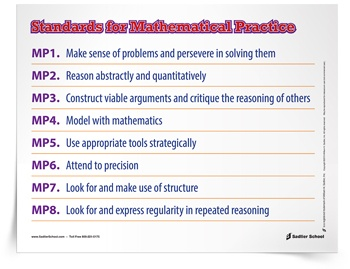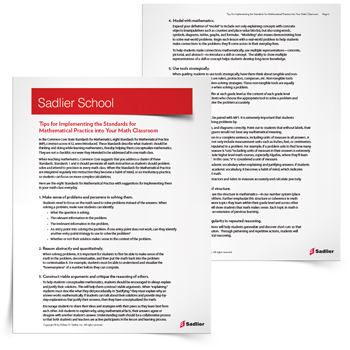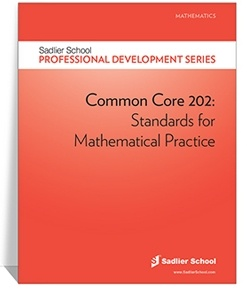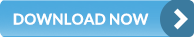1.800.221.5175
Core Program
Full Access
Core Program
Full Access
Core Program
Full Access
National Program
Full Access
New York
Critical Thinking for Active Math Minds
Preparing for Standards Based Assessments
Vocabulary Workshop, Tools for Comprehension Grades 1–5
Print Program
Interactive Edition
Print Program
Interactive Edition
Vocabulary Workshop, Tools for Excellence Grades 6–12+
Print Program
Interactive Edition
Vocabulary for Success
Progress English Language Arts Grades K–8
National Program
Full Access
New York
Grammar Workshop, Tools for Writing
Grammar for Writing
Writing a Research Paper
Writing Workshop
Print Program
Interactive Practice Bundle
Fluency Booster Practice Book
Print Program
Interactive Edition

A K–8 resource to support deep comprehension of math skills and conceptsTopics:

#### April 21, 2016 k-2-math-practices, 6-8-math-practices, mp-problem-solving, 3-5-math-practices

Making sense of problems and persevering in them is Mathematical Practice 1, a part of the Common Core Mathematical Practices. This post covers this standard and how it can be simplified for grades K–5 and 6–8. At the end of the post, you’ll find downloadable posters in student-friendly language that you can display in your classroom.The Eight Common Core Mathematical Practices are the ways that students should think about math and solve problems. Although they do not include the mathematical content that we teach, they are useful standards for the reasoning that students can use while we teach about content. They originate from two previous sets of standards: 1) the process standards created by the National Council of Teachers of Mathematics, and 2) the strands of mathematical proficiency included in the report Adding It Up by the National Research Council.

Mathematical Practice 1 is one of two overarching Common Core Mathematical Practices. Along with Mathematical Practice 6 (attending to precision), it serves as a way for students to structure their thinking and communication about a problem they need to solve. Making sense of problems and persevering in solving them looks different at the lower and middle grade levels than at the high school level. We will first look at adapting them for grades K–5, then for grades 6–8.

To adapt the Common Core Mathematical Practices for the lower grades, Illustrative Mathematics (February 2014) wrote “elaborations” of them. These adaptations are more developmentally appropriate for younger students and are geared toward the types of math they are learning. The version of MP1 for the early grades follows. Notice that the practice is applied to early grade math by focusing on counting, base-ten numbers, place value, and operations. The last sentence is one of the keys — students should show flexibility in their learning by understanding how other students’ solutions relate to their own.

## Make sense of problems and persevere in solving them.

Mathematically proﬁcient students at the elementary grades explain to themselves the meaning of a problem, look for entry points to begin work on the problem, and plan and choose a solution pathway. For example, young students might use concrete objects or pictures to show the actions of a problem, such as counting out and joining two sets to solve an addition problem. If students are not at ﬁrst making sense of a problem or seeing a way to begin, they ask questions that will help them get started. As they work, they continually ask themselves, “Does this make sense?" When they ﬁnd that their solution pathway does not make sense, they look for another pathway that does. They may consider simpler forms of the original problem; for example, to solve a problem involving multi digit numbers, they might ﬁrst consider similar problems that involve multiples of ten or one hundred. Once they have a solution, they look back at the problem to determine if the solution is reasonable and accurate. They often check their answers to problems using a diﬀerent method or approach.

Mathematically proﬁcient students consider diﬀerent representations of the problem and diﬀerent solution pathways, both their own and those of other students, in order to identify and analyze correspondences among approaches. They can explain correspondences among physical models, pictures or diagrams, equations, verbal descriptions, tables, and graphs. (p. 12)

Similarly, they adapted MP1 for the middle grades (Illustrative Mathematics, May 2014). The middle school version of this Common Core Mathematical Practice is below. Notice how it applies the same ideas to middle grade content. The focus at the middle grades is on ratios, tables, graphs, and expressions.

## Make sense of problems and persevere in solving them.

Mathematically proﬁcient students set out to understand a problem and then look for entry points to its solution. They analyze problem conditions and goals, translating, for example, verbal descriptions into mathematical expressions, equations, or drawings as part of the process. They consider analogous problems, and try special cases and simpler forms of the original in order to gain insight into its solution. To understand why a 20% discount followed by a 20% markup does not return an item to its original price, they might translate the situation into a tape diagram or a general equation; or they might ﬁrst consider the result for an item priced at \$1.00 or \$10.00. While working on a problem, they monitor and evaluate their progress and change course if necessary.

Mathematically proﬁcient students can explain how alternate representations of problem conditions relate to each other. For example, they can navigate among tables, graphs, and equations representing linear relationships to gain insights into the role played by constant rate of change.

Mathematically proﬁcient students check their answers to problems and they continually ask themselves, “Does this make sense?” They can understand the approaches of others to solving complex problems and compare diﬀerent approaches. (p. 12)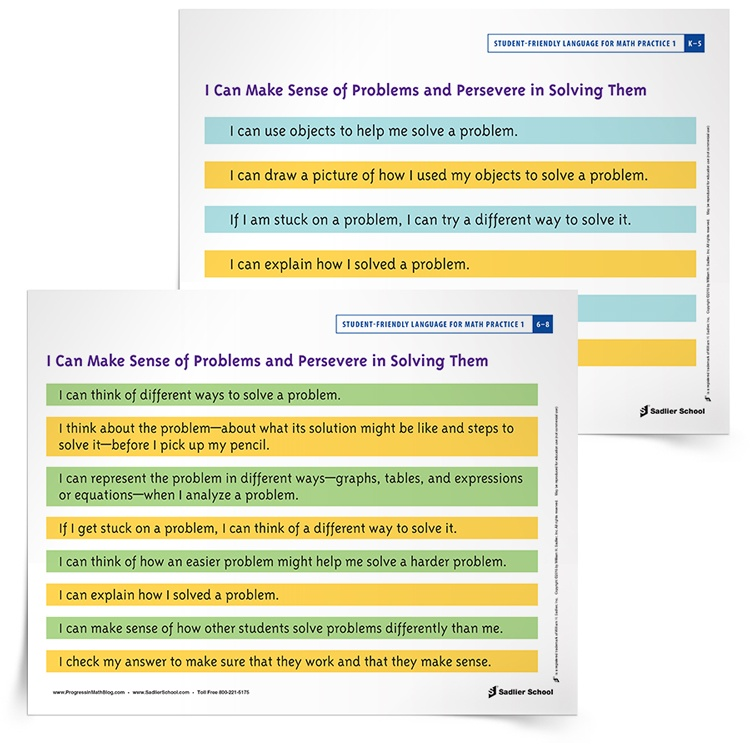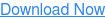For your students to use Mathematical Practice 1 from the Common Core Mathematical Practice, download the posters for grades K–5 and grades 6–8. These posters use student-friendly language to break down what it means to Make Sense of Problems and Persevere in Solving Them for your students.